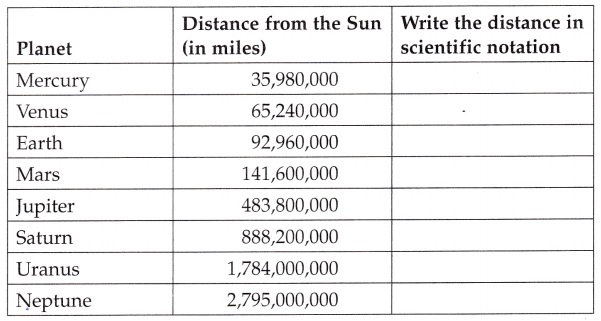# McGraw Hill Math Grade 8 Lesson 10.8 Answer Key Estimation and Comparison

Practice the questions of McGraw Hill Math Grade 8 Answer Key PDF Lesson 10.8 Estimation and Comparison to secure good marks & knowledge in the exams.

## McGraw-Hill Math Grade 8 Answer Key Lesson 10.8 Estimation and Comparison

Exercises

Use this chart to answer the questions.Question 1.
Write each distance in the chart in scientific notation.Explanation:
Scientific notation is a way to make these numbers easier to work with.
In scientific notation, you move the decimal place until you have a number between 1 and 10.
Then you add a power of ten that tells how many places you moved the decimal.
In scientific notation, the distance between the sun and planet Mercury is 35,980,000 miles becomes 3.598 x 107
The distance between the sun and planet Venus is 65,240,000 miles becomes 9.296 x 107
The distance between the sun and planet Earth is 92,960,000 miles becomes 3.598 x 107
The distance between the sun and planet Mars is 141,600,000 miles becomes 1.416 x 108
The distance between the sun and planet Jupiter is 483,800,000 miles becomes 4.838 x 108
The distance between the sun and planet Saturn is 888,200,000 miles becomes 8.882 x 109
The distance between the sun and planet Uranus is 1,784,000,000 miles becomes 1.784 x 109
The distance between the sun and planet Neptune is 2,795,000,000 miles becomes 2.795 x 109

Question 2.
How many times larger is Uranus than Saturn?
2 times larger
Explanation:
Distance of Uranus – 1784,000,000 = 17.84 x 107
Distance of Saturn – 888,200,000 = 8.882 x 107
8.8 x 2 = 17.6 2 times larger
Uranus is two times  larger than Saturn.

Question 3.
How many times larger is Jupiter than Earth?
5 times larger
Explanation:
Distance of Jupiter 483,800,000 = 48.38 x 107
Distance of Earth 92,960,000 = 9.296 x 107
9.296 x 5 = 46.48
Jupiter is 5 times larger times larger than Earth.

Question 4.
Estimate the distance from the Earth to the Sun in scientific notation.